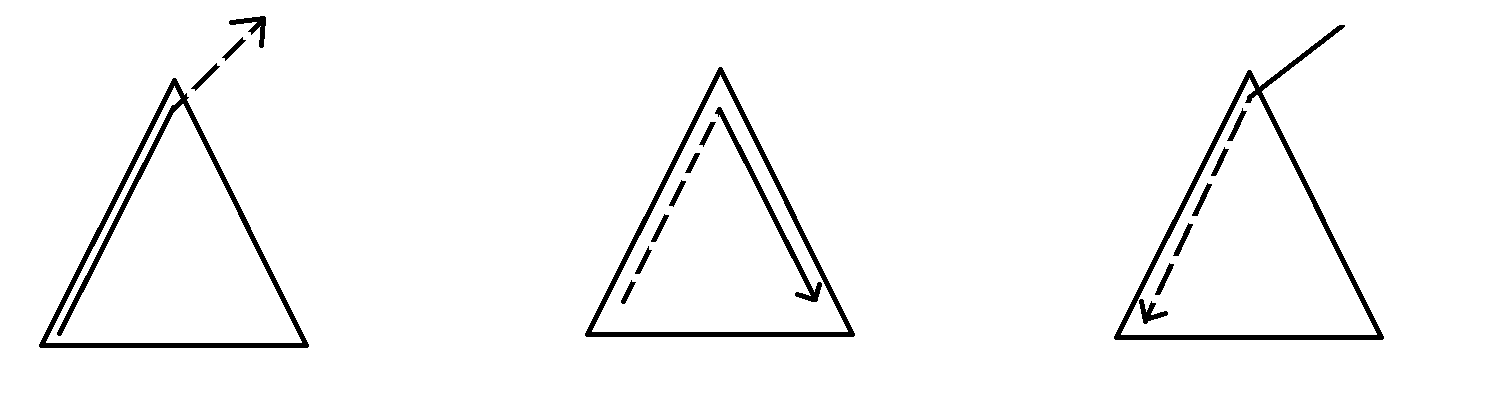# arrow_back动态规划共96篇文章zc
2019-12-21 19:47:00zc
2019-12-21 19:47:00

f[i][k]为第i位,分成k

f[i][k] = MAX(f[j][k-1] + cnt[j+1][i])zc
2019-12-21 19:47:00

f(i,j)表示前i个划分成j段最少的费用

f(i,j)=\min_{x=1}^i(f(x-1,j-1)+calc(x,i))

i<i',那么k_i\le k_{i'}(决策点)zc
2019-12-21 19:47:00

f[x]表示状态为x时最少走多少步

f[x|2^{i-1}|2^{j-1}]=\min(f[x|2^{i-1}|2^{j-1}],f[x])

(如果不懂可以自己模拟一下)zc
2019-12-21 19:47:001. x子树内\rightarrow x \rightarrow x子树外(包括x)

2. x子树内(包括x)\rightarrow x \rightarrow x子树内

3. x子树外\rightarrow x \rightarrow x子树内

1. ans += V_x \times (f_x-g_x) \times (n-siz_x+1)(可以在x的子树中随机选一个往子树外面走(可以指走到x))

2. ans += V_x \times (g_{to}-f_{to}) \times (siz_x-siz_{to}-1)

ans+= V_x \times (siz_x-1)(可以从x直接向下走)

3. 我们需要记录x子树外与x的距离为偶数和与x的距离为奇数的点的个数,分别记作uf_x,ug_x

我们可以通过bfs从上到下来处理,那么

uf_x=ug_{fa_x}+g_{fa_x}-f_x

ug_x=uf_{fa_x}+f_{fa_x}-g_x

ans += V_x \times (uf_x-ug_x) \times siz_xzc
2019-12-21 19:47:00

## 题目大意：

\forall l\in\left[1,n\right]:a_l \leq a_r + f_{r} - \sqrt{|r-l|}

## 解法

f_r= \max(a_l+\sqrt{r-l})-a_r(l\in[1,r])

Search
search